Latest Banking jobs   »

# Reasoning Quiz For RBI Grade B Phase 1 2023 -05th May

Directions (1-5): Study the information carefully and answer the questions given below.
Ten persons are sitting in a row some are facing north while some are facing south. E sits fourth to the right of D who sits one of the extreme ends of the row. More than two persons are sits between K and E. B sits third to the left of C who is an immediate neighbour of K, both are facing same direction. A sits fourth to the left of the one who sits immediate right of B. H sits third to the right of the one who sits second to the left of F who is not an immediate neighbour of B. Both H and E facing opposite direction to each other. I sits fourth to the right of G, both are facing opposite direction. H sits third to the right of G. Persons who sits at extreme ends faces opposite directions. K does not face north. A and I facing same direction.

Q1. How many persons are sitting between A and C?
(a) Two
(b) Three
(c) None
(d) Four
(e) More than four

Q2. Who among the following sits third to the left of B?
(a) H
(b) C
(c) G
(d) A
(e) None of these

Q3. What is the position of E with respect to G?
(a) Immdiate right
(b) third to the left
(c) Second to the right
(d) Immdeate left
(e) None of these

Q4. Which of the following is true about K?
(a) K sits third to the right of B
(b) Only four persons sit between K and G
(c) K facing same direction as E
(d) H sits third to the left of K
(e) None is true

Q5. Four of the following are alike in a certain way so form a group, who among the following does not belong to that group?
(a) G
(b) C
(c) H
(d) F
(e) D

Directions (6-7): Read the following information carefully and answer the given questions:
P + Q means P is the sister of Q
P # Q means P is the husband of Q
P \$ Q means P is the daughter of Q
P % Q means P is the mother of Q
P @ Q means P is the brother of Q

Q6. What should come in place of the question mark, to establish that B is the child of K in the expression?
T % K # F % L + N ? B
(a) Only %
(b) Either + or @
(c) Only #
(d) Either @ or %
(e) Only +

Q7. Which among the given expressions indicate that J is the wife of F?
(a) P \$ J % R + K % F
(b) F @ R + K + P % J
(c) J % P + K @ R \$ F
(d) J % P # K + R + F
(e) None of these

Directions (8-9): Study the following information carefully to answer the given questions:
There are six persons viz. A, B, C, D, E and F of different ages. C is older than only A and E. D is younger than only B. E is not youngest. The one who is third oldest, age 81 year. E’s age is 62 years.

Q8. Which of the following could possible age of C?
(a) 70 years
(b) 94 years
(c) 86 years
(d) 61 years
(e) 81 years

Q9. Which of the following is true with respect to the given information?
(a) D’s age is definitely less than 60.
(b) F is oldest.
(c) Only two people older than C.
(d) There is a possibility that B’s age is 79 years
(e) None is true.

Q10. Mohan walked 40km towards North and then he took a left turn and walked 20km. He again took a left turn and walked 40km. How far and in which direction is he from the starting point?
(a) 30km west
(b) 10km North
(c) 20km South
(d) 15km South
(e) 20km west

Directions (11-12): Study the following information carefully and answer the given questions:
A person starts walking from point A to the east direction and walks 24m to reach at point B. From point B he takes two consecutive left turns and walks 10m and 12m respectively and reach at point D. From point D he walks in south direction and walks 15m to reach at point E, then finally turn his left and walks 12m to reach final point F.

Q11. What is the direction of his starting point with respect to point D?
(a) North-west
(b) South-West
(c) North-east
(d) South
(e) None of these

Q12. If point O is the midpoint of point A and B, then what is the shortest distance between point O to his final position?
(a) 15m
(b) 12m
(c) 10m
(d) 13m
(e) None of these

Directions (13-15): Study the following information carefully and answer the given questions:
Point A is 20m west of point B, which is 9m north of point D. Point F is 6m south of point G. Point E is 15m north of point C. Point F is 18m east of point E. Point C is 12m west of point D.

Q13. What is the direction of point G with respect to point D?
(a) South
(b)North-east
(c)South-west
(d)East
(e) None of these

Q14. What is the shortest distance between point E to point A?
(a)12m
(b)18m
(c) 25m
(d) 10m
(e) None of these

Q15. What is the direction of point A with respect to point C?
(a) North-West
(b)South-East
(c)North-East
(d) West
(e) None of these

Solutions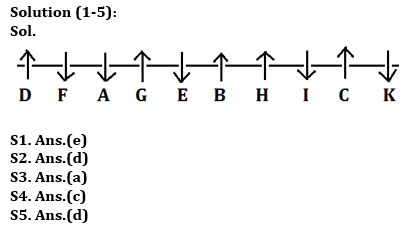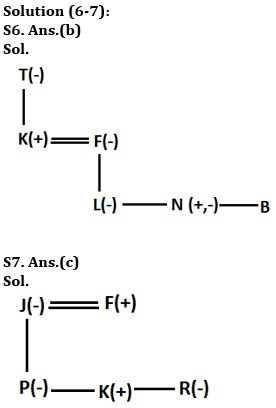Solution (8-9):
Sol. According to their age= B > D > F (81) > C > E (62) > A

S8. Ans.(a)
S9. Ans.(e)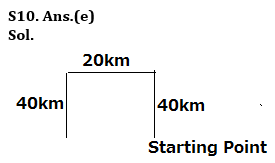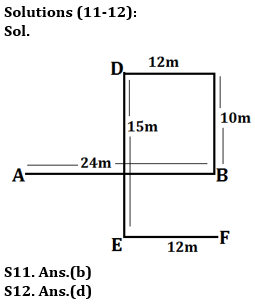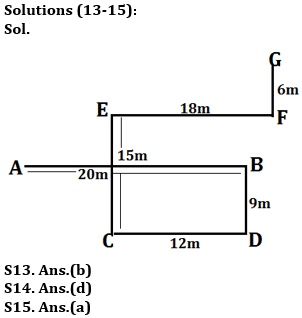## FAQs

### What is the selection process of the Bank Clerk?

There are 4 sections in the RBI Grade B Phase 1 Exam i.e. English Language, General Awareness, Quantitative Aptitude & Reasoning.

#### Congratulations!Union Budget 2023-24: Free PDF